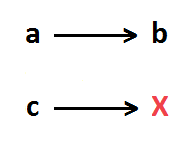# Single rule of three direct online

Find out x, in such proportion to c as b is to a.For example:

If 4 kg of a product cost \$36, how much would be the cost of 18 kg? Answer: a: 4, b: 36, c: 18, x: 162 (\$162)

If 2 liters of paint are required to paint 5 rooms, how many liters are required to paint 7 rooms? Answer: a: 5, b: 2, c: 7, x: 2.8 (liters)

#### Options

• Value of a
• Value of b
• Value of c
• Number of decimal places (round)